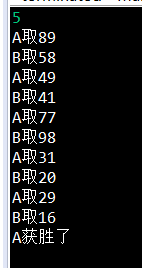算法学习——贪心算法之取数游戏（显示两端数字）

算法描述

A的取数策略：采用贪心策略，每次取数取两个数中最大的那个数

B的取数策略：当两个数相差较大，取大的那个数，若相差为1，则在这两个数中随意取一个数

算法思路

1. 随机产生数值，我们使用Java中的Math.random()方法即可

2. 为了方便取数，将随机产生的2n个整数放在链表中，链表中有方法可以取出第一个数和最后一个数

getFirst()返回第一个元素的数值，不将该元素移出链表

removeFirst()将第一个元素链表链表，返回该元素的数值

getLast()返回最后一个元素的数值，不将该元素移出链表

removeLast()将最后一个元素移出链表，返回该元素的数值

3. 设置一个布尔变量flag,flag=true轮到A取数flag=false轮到B取数

4. 分别用两个一维数组存放A与B的取数

5. A取数采用贪心策略，我们使用get方法，获得一个数和最后一个数，取大数存放在A数组中

6. B取数有两个选择，相差较大（也就是相差大于1），我们就取大数（这里我们在实际当中需要采用绝对值）;

若是等于1，则随意取，我们可以使用随机数的方法，若是随机数大于0.5，则取第一个数，否则取末尾那个数

如果是A先取数的话，B到最后会出现只剩下一个数的情况，这个时候B没有选择，只能取这个数

7. 判断输赢的话，只需要比较A数组的总和与B数组的总和即可判断出输赢

算法实现

Scanner scaner = new Scanner(System.in);
int n = scaner.nextInt();
scaner.close();
int[] A = new int[n];//存放A所取的数值
int[] B = new int[n];//存放B所取的数值

for(int i=0;i<2*n;i++){
int temp=(int)(Math.random() * 100) ;//0到100的随机数
}

boolean flag = true;//A先取数
int count = 0;
//当B数组的最后一个不为0的时候（即是B拿到了最后一个数的时候），退出while
while(count<n){
if(flag){

if(list.getFirst()>list.getLast()){
A[count] = list.removeFirst();
}else{
A[count]=list.removeLast();
}
System.out.println("A取"+A[count]);
flag=false; //改变flag，轮到B取数
}else{

if(list.getFirst()==list.getLast()){//当还剩下一个数的时候，B只能取当前那个数
B[count]=list.removeFirst();
}else if(list.getFirst()-list.getLast()>1){
B[count] = list.removeFirst();//选择最大的那个数
}else if(Math.abs(list.getFirst()-list.getLast())==1){
//B随机选择
if(Math.random()>0.5){
B[count]=list.removeLast();
}else{
B[count]=list.removeFirst();
}
}else{
B[count]=list.removeLast();//第一个数比末尾的数要小，因为上述条件已经排除了等于1的情况，所以末尾的数一定是与第一个数相差较大
}
System.out.println("B取"+B[count]);
count++;
flag = true;//轮到A取数
}
}

int a=A,b=B;
//判断输赢
for(int i=1;i<A.length;i++){
a = a+A[i];
b = b+B[i];
}

if(a>b){
System.out.println("A获胜了");
}else{
System.out.println("B获胜了");
}

结果posted @ 2018-10-27 16:56  Stars-one  阅读(2525)  评论(0编辑  收藏  举报